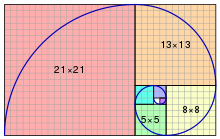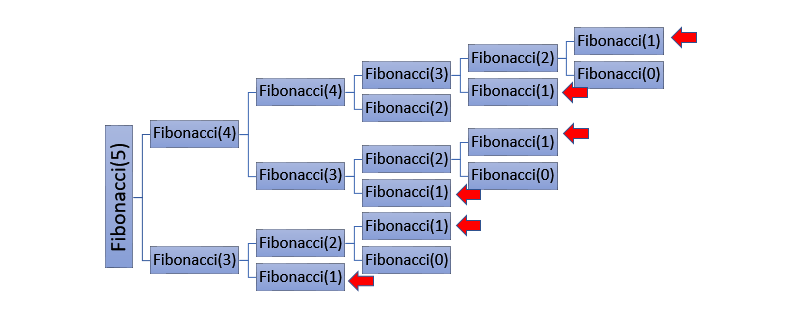top of page
Search
• Eric Young

# Algorithms in Real Life: Space v.s. Time

Updated: Jul 18, 2021

In Computer Science, we are often challenged to “do better” ― even though our program works, we are asked to make it run faster or use up less space. Since there are often different ways to implement a solution to the same problem, different algorithms that achieve the same goal can yield wide-ranging time and space complexities. Why might the running time of an algorithm matter? Simply put, an algorithm is ultimately useless if it takes forever to run ― we never get our desired output. Why might the space that an algorithm takes up matter? Space can be expensive sometimes. However, as memory is becoming cheaper and cheaper with advancements in technology, programmers are usually willing to trade more space for a faster runtime. Let me illustrate the tradeoffs between time and space complexity by analyzing two different ways of solving the Fibonacci problem.

The Fibonacci Problem:

The Fibonacci Sequence is a famous series of numbers shown below:

0, 1, 1, 2, 3, 5, 8, 13, 21, 34, 55, 89, 144, 233, 377, 610, 987, 1597, 2584

As you can see, the first number is 0, the second number is 1, and the next numbers are the sum of the two numbers before it:

1. The 2 is found by adding the two numbers before it (1+1),

2. The 3 is found by adding 1+2,

3. The 5 is found by adding 2+3,

4. And so on…

When we try to picture the Fibonacci Sequence using squares, we see a nice spiral! 5 and 8 make 13, 8 and 13 make 21, and so on…Turns out this spiral property of Fibonacci sequences are also seen prevalently in real life, especially in nature.We are now tasked to solve the Fibonacci problem: Given any positive integer n, find the nth number in the Fibonacci Sequence. We could, of course, calculate this by hand. If we want to find the 5th Fibonacci number, we could just start from the first two Fibonacci numbers and follow the definition until we get to the 5th term: 0, 1, 1 (0+1), 2 (1+1), 3 (1+2). However, this start-from-scratch method quickly becomes impractical as n gets large ― it would be a huge waste of time for us to manually calculate the 1000th Fibonacci number! Thus, we turn to a computer to help us solve this problem.

Naive Recursive Solution:

Using Python (or another programming language), we can implement a simple recursive program that computes the nth Fibonacci number.For an input of 5 to our naive recursive solution, we can observe the pattern above. In a tree-like structure, fib5 (fib_rec(5)) is broken down into fib4 and fib3, fib4 is broken down into fib3 and fib2, fib3 is broken down into fib2 and fib1, and finally fib2 is broken down to our base cases: fib1 and fib0, which evaluate to 1 and 0 respectively. The recursive algorithm then uses our known base case values to compute all the other subproblems (e.g. fib2, fib3, etc…) in a bottom up approach. It is important to note that without our base cases specified, our algorithm would not work because there would be no stopping point. In this case, a stack overflow error would be thrown.

Scanning the diagram from top to bottom, we notice that as we move downwards, each layer of fib items grows exponentially in size. Layer 0 has 1 (2^0) item,  layer 1 has 2 (2^1) items, layer 2 has 4 (2^2) items, layer 3 has the potential for 8 (2^3) items, layer 4 has the potential for 16 (2^4) items, and layer n has the potential for 2^n items. Since adding more inputs means adding more fib layers with items growing exponentially, the time complexity of our solution is O(2^n). This turns out to be very inefficient for even not-so-large input sizes like 50 or 100. For example, while fib_rec(40) takes a few seconds to run, fib_rec(80) takes theoretically years to run. While it is still feasible to solve small Fibonacci numbers with our naive recursive solution, we cannot solve large Fibonacci numbers using this approach.

Dynamic Programming Solution:

Can we do better?

When brainstorming for more optimal solutions, we can begin by analyzing the flaws of our less optimal solution. In the recursive tree diagram, we notice that there are a lot of overlapping computations. For instance, fib2 is repeatedly calculated 3 times and fib3 is repeatedly calculated 2 times. While this may not seem like a lot, the exponential increase of overlapping computations will add up when we have larger inputs, such as 50 or 100. What if we store the results of these repeated computations somewhere? Then, we can use them directly instead of recomputing when we need them. This “memoized” (memo = a note recording something for future use) solution is a common problem-solving strategy known as Dynamic Programming (DP).Before we define the function fib_dyn(n), we first create an array with null (empty) values that acts as a cache, or a structure that stores data so that future requests for that data can be served faster. Trading space for speed, we store the value of fib0 at index 0 of the cache array, fib1 at index 1, fib2 at index 2, etc… To compute a new fib value, we just need to look up the previous two entries in the cache array. With our base cases defined, we can simply use cache and cache to find cache, cache and cache to find cache, etc…, successfully avoiding the unnecessary overlapping computations. The time complexity for our DP solution is O(n) (linear) for traversing the cache array, which is significantly faster than our O(2^n) (exponential) recursive solution. The only downside of our DP solution is the added space complexity of O(n) for creating the cache array. This downside, however, is minuscule compared to the amazing benefit of a drastically reduced time complexity. In fact, I was able to run fib_dyn(1000) and get the accurate result almost instantly.Conclusion:

Here are several key takeaways from this post:

1. Programmers care about how fast a program runs and how much space it takes up because these attributes dramatically impact user experience.

2. When looking for a more optimal solution, examine the flaws of the less optimal solution and identify patterns. Find a way to get around the flaws (with perhaps a new data structure), and develop your improved solution from there.

3. Trading space for speed is encouraged in most situations.